# Lesson 4: Excel Data Tables and Monte Carlo Simulations

Introduction

Today, I'm going to tell you something about Monte Carlo simulations within Excel.

Monte Carlo? Let's go gambling baby!

Well, you're right, that is where the name comes from. Monte Carlo simulations allow us to assess the impact of certain parameters in a forecasting model. I'll give an example.

Suppose you're manufacturing some goods. On one hand, the cost of these goods depends on the cost of the raw materials you need to purchase and the manpower needed to manufacture these goods. On the other hand, you'll export all these items abroad and depending of the exchange rate your activity will be more or less profitable.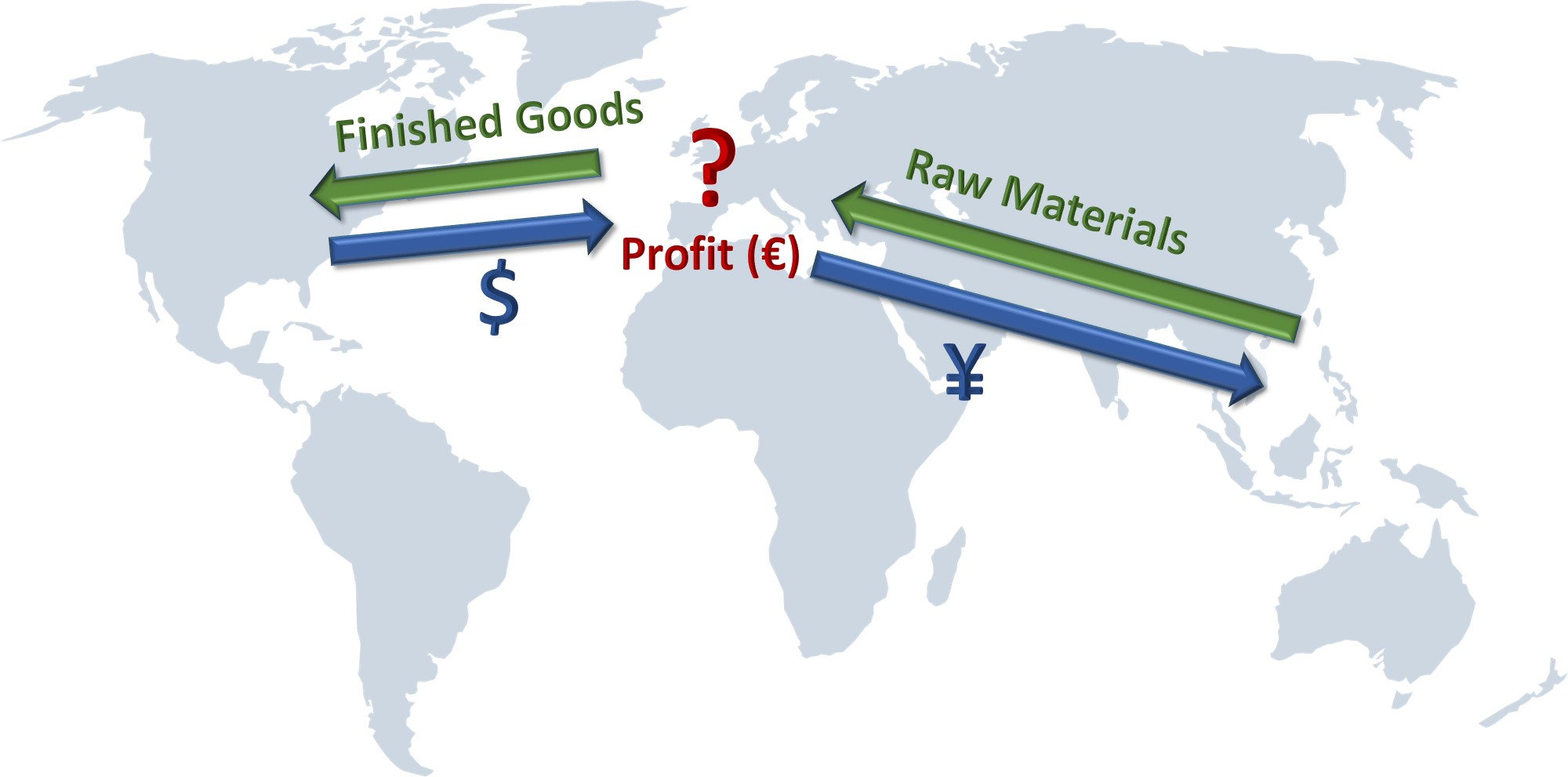You've put your accountants, treasurers, procurement officers and controllers at work and they have built a model telling you how the price of raw materials and exchange rates are likely to evolve in the future. The word likely is key here. It's about statistics. You don't know how things are going to evolve but you have an idea of how they might evolve with some probability attached. Given this, Monte Carlo will run scenarios against your model, changing the inputs according the variability you defined and measuring the results afterwards. So, instead of giving you just one final figure, Monte Carlo will provide you with a range of values as a result and how likely the resulting outcomes are. Isn't that great?

So, it will help me to make decisions or mitigate my risks.

For sure! But before showing how Monte Carlo can be used in Excel, I need to tell you something about a really powerful Excel tool: Data Tables.

Excel Data Tables

Let's have a closer look at the example below. You're a Europe based company that buys some raw materials in Japan at a certain exchange rate, manufactures them to some finished goods and sells them in the United States of America again taking into account a certain exchange rate.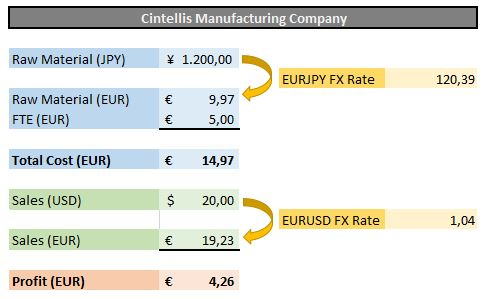Hold on! I don't care about the business model, it is just an example to make things clear. Now, your company treasurer or your financial controller would like to estimate, ceteris paribus, what the profit will be if the EURJPY rate changes over time.

Easy! Just change the FX rate value and Excel will recalculate everything automatically.

Well you could do that, but I suppose you'd like to have an overview of the profit evolution.

Copy and paste the result each time and compare it afterwards will do the trick I guess.

Yes it will, but it will as well be a waste of your time. I'm sure you've better things to do, right? Don't feel obliged to answer that question...

Whatever, this is where Data Tables can be useful. First you need to provide a list of EUR vs JPY exchange rates you'd like your profit to be calculated with. This is the first column in the table below. In this example, I want to know my profit if the FX rate varies between 110 and 130. In the top cell of the second column, you need to refer to the cell you want to watch, in this case the profit in cell D15.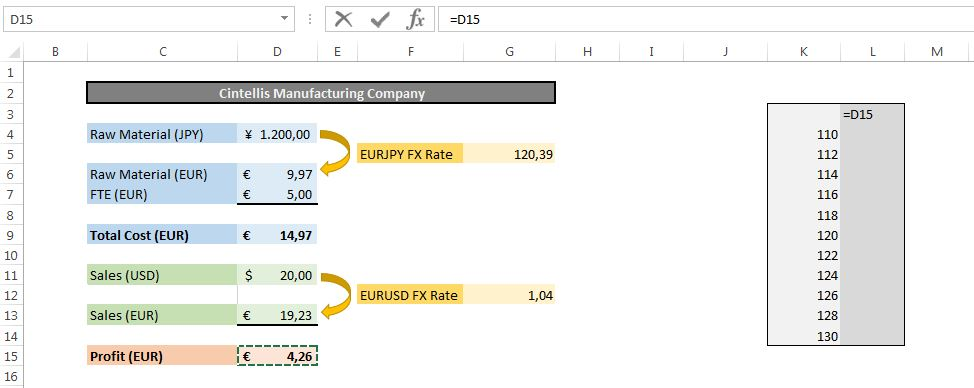We're now all set to let Excel (and the Data Tables) do the computing for us. We need to select the table in grey we just created and go to the Data Table section of the "What-If Analysis" tab in the ribbon: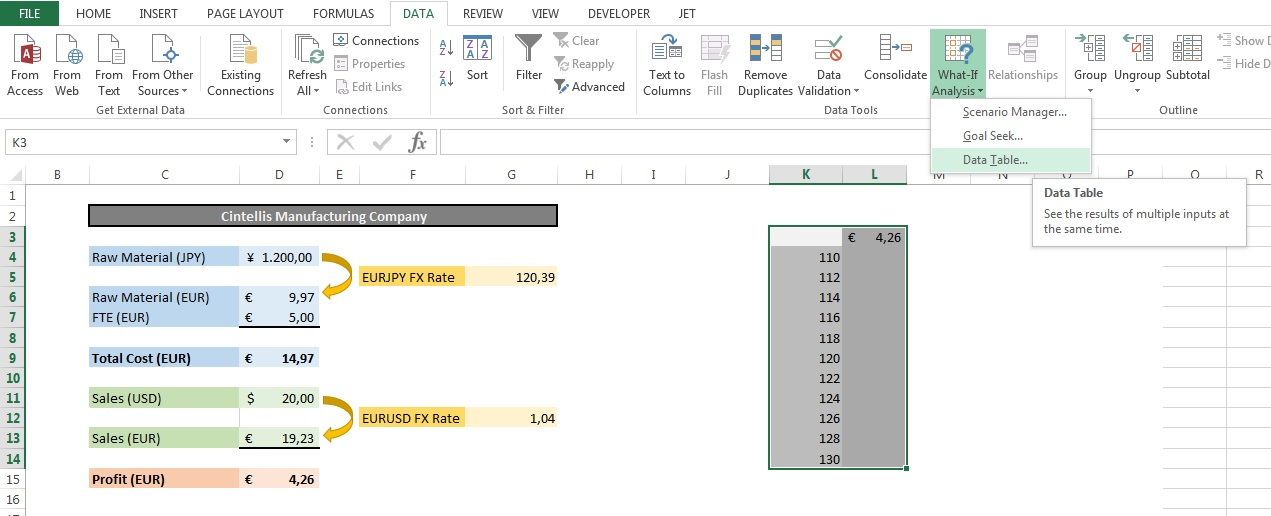Excel then asks you the following question: "You provided me a lot of values in that first column, but to what parameter (i.e. cell) does it refer to in your model?"

I guess that would the EURPJY exchange rate in cell G5?

Correct. So as your variable data has been stored in columns you need to put G5 in the column input cell field: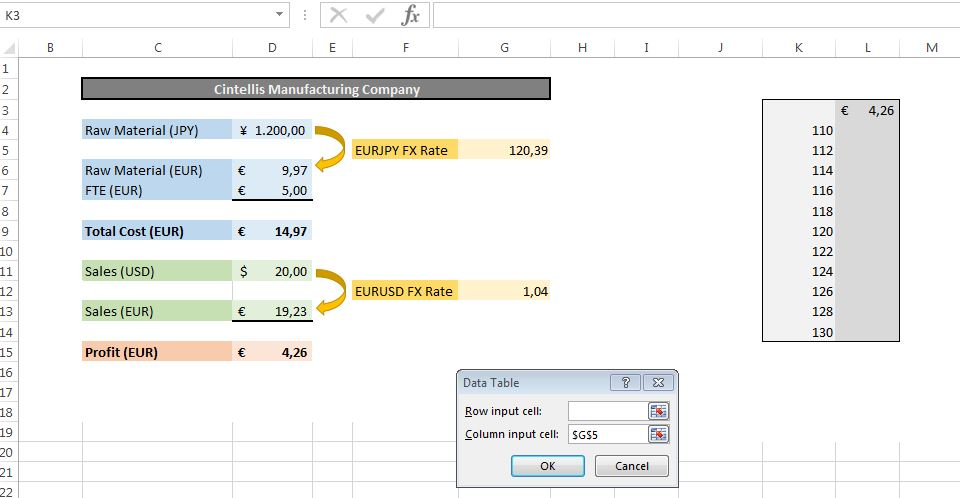What follows next is pure magic there Excel will do all the iterations for you and will tell you what your profit will be for each FX rate: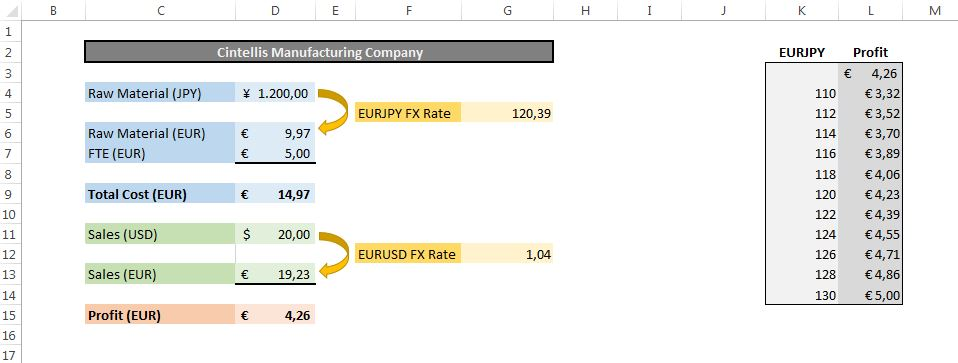And what if my EURUSD rate changes as well?

Two-Dimensional data tables

You can barely walk and would like to run already! Anyway, the answer to your question is however an easy one: you can create data tables with two dimensions: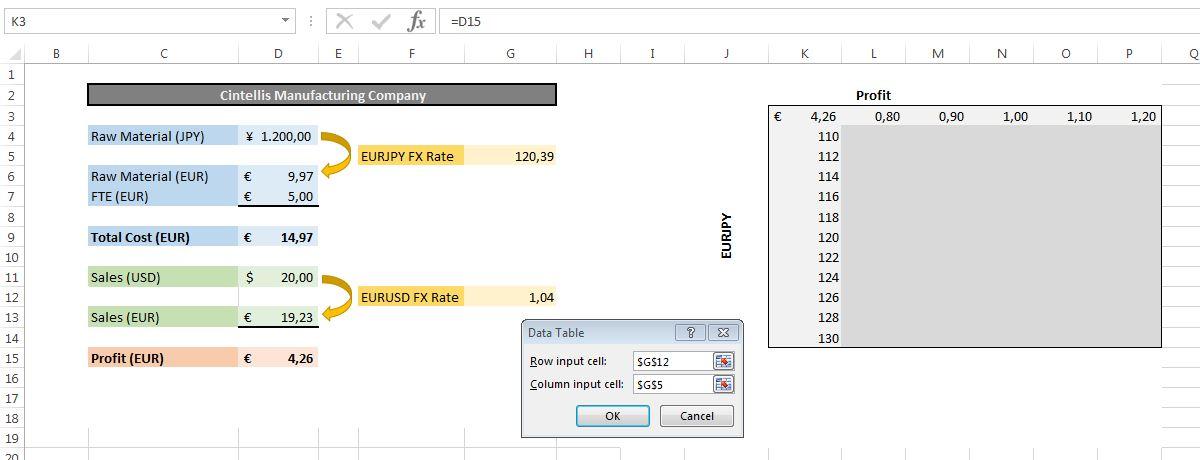As shown above, you should now tell Excel the EURJPY rate is in the table column, whereas the EURUSD rate is organized in rows. Note that the cell to watch (our profit) is now at the intersection of the row and column containing the exchange rates.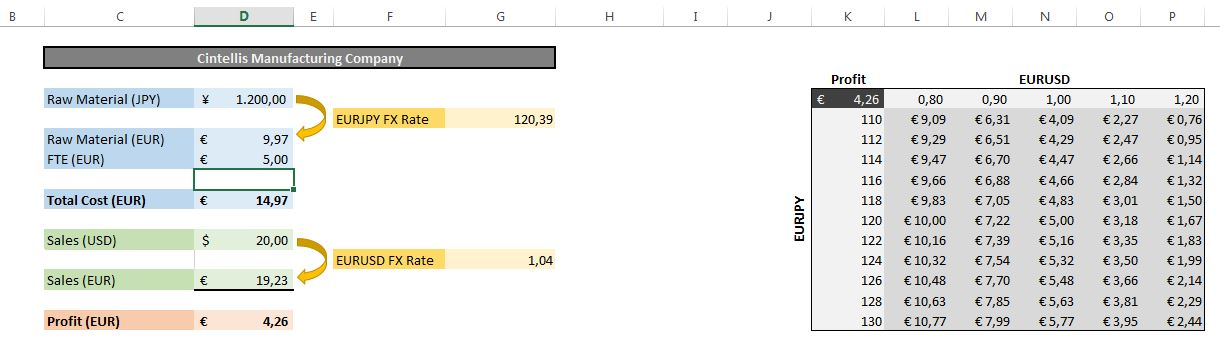So, this table tells me that if the JPY strengthens against the EUR to 110 and the EURUSD hits a 1,20 rate, my profit will drop to 0,76ï¿½, correct?

Absolutely.

As I understood, it seems I'm ready for the next step already. I want a third variable in my model, there the FTE cost could change as well. (Dear reader, if you saw the same as I did, he could only set 2 variables in his data table model and not any more. Let's make our specialist sweat now...)

You want a third variable? No problem. I propose to add as well another variable to watch, like the total cost in EUR, how does that sound?

Damn, you can do that?

Multi-dimensional data tables - Scenarios

The way to deal with multiple input variables is to define upfront the scenarios you would like to assess: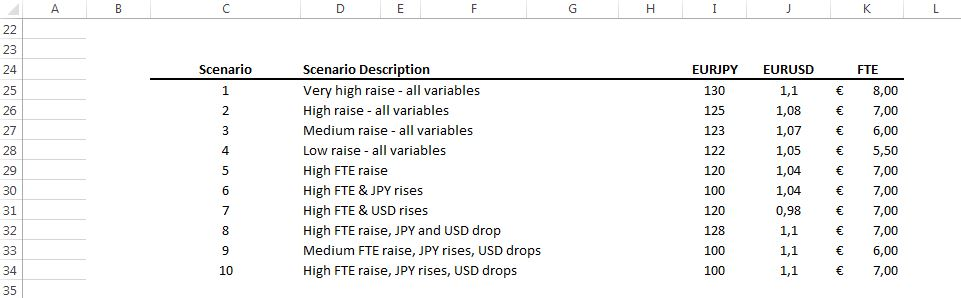Choose then a random cell in which you'll select what scenario you want to see in your model. You'll need to link the model to the scenarios using i.e. VLOOKUP's, as for instance can be seen below for the EURJPY FX Rate: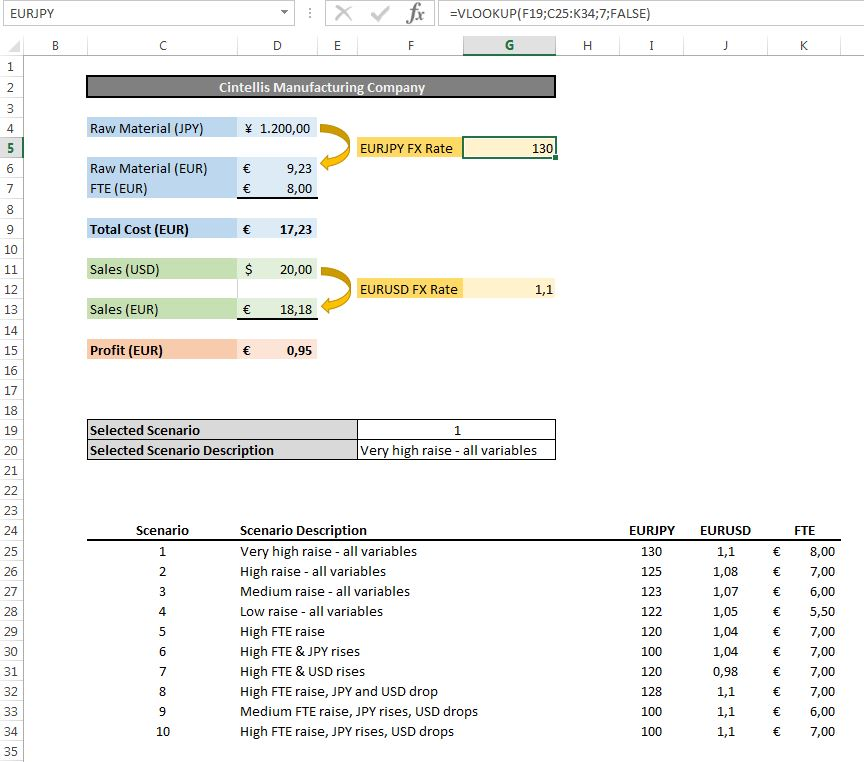OK, so if I now modify cell F19, my model will use the values of all variables according the chosen scenario.

That is correct.

Hold on, I know! You'll create a data table where you'll iterate on all your predefined scenarios. So basically it just becomes a data table with one single input parameter being the scenario number.

But how about our multiple output variables? We need to see the impact on both the Profit and the Total Cost.

Well, in a model with just one input parameter, you can create as many output columns as you want like shown below: the first column refers to cell F20, the scenario description. The second column refers to cell D9, our total cost and the last one to cell D15, the profit.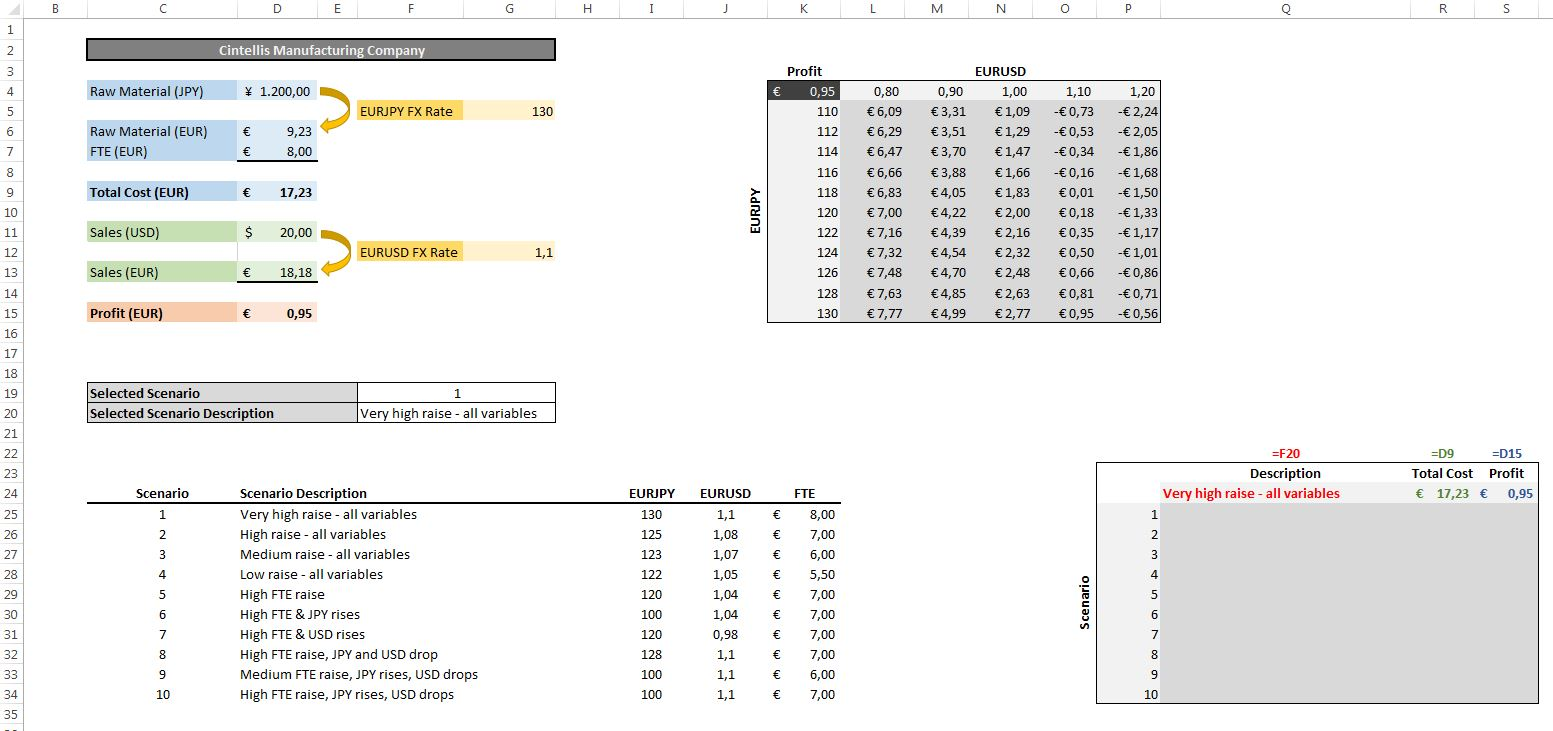Select afterwards your table and choose the cell with the selected scenario (F19) as input column. Excel will then for each scenario number modify cell F19 which in turn will affect the values in our model. The result will be shown in the data table: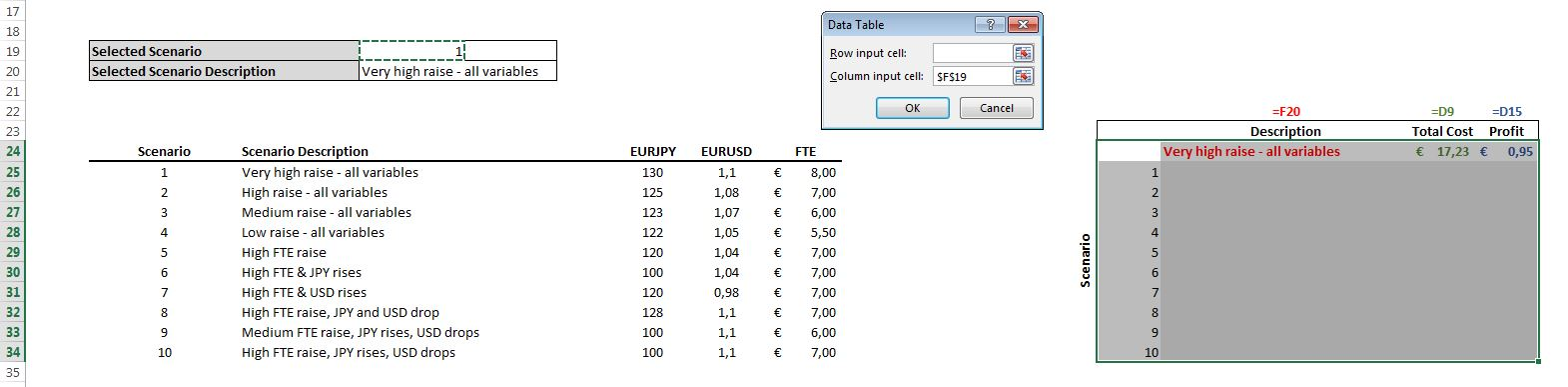When do we start gambling?

Monte Carlo Simulations

What is now going to change in comparison to the above is that we'll add some statistics to our model. Suppose you've studied the past 10 years of data and you've come to the conclusion that the cost of labor follows a normal (or Gaussian, bell shaped around a mean) distribution. The mean appears to be 6,00 ï¿½ and the standard deviation is 0,70 ï¿½.

Let me explain the statistics, I want our readers to understand. What the above situation tells us is that on average, the cost of labor was 6,00 ï¿½, and you had 68% chance that the cost was within one standard deviation away from the mean, hence in the range 5,30 ï¿½ - 6,70 ï¿½. There was about 95% chance that the value lies within two standard deviations and about 99,7% were within three standard deviations.

Important is now to assume that this as well will be the way the cost of labor will behave in the future. Let's now put our model at work. Please by my guest...

I don't even know where to start!

What have we been doing all the time?

We worked with data tables. Hold on, I think I just saw the light. Basically, you want to know what the profit will be if the cost of labor varies according the above statistical model. And that is what data tables can be used for.

Correct.

But we've always used fixed values. How do I tell Excel that my cost of labor will follow a particular statistical distribution?

Excel has plenty of statistical features that are really handy. The one we will need here is the NORM.INV function. NORM.INV takes three input parameters:

• a probability P;
• a mean M;
• a standard deviation S.

Excel will then, given a Gaussian distribution of mean M and standard deviation P, return a value such that the probability that you'll hit a number that is less than or equal to that value has a probability of P. So Excel will return exactly 5 for the function "=NORM.INV(0,5;5;2)" there in a Gaussian distribution you've exactly 50% chance of hitting a number that is less than or equal to the mean. If you now use "=NORM.INV(0,6;5;2)", Excel will return 5,507 there again, in such a Gaussian distribution you've 60% chance of hitting a number that is less than or equal to 5,507.

Yes, but for Monte Carlo, I need a random number for my cost of labor.

Look at the example below: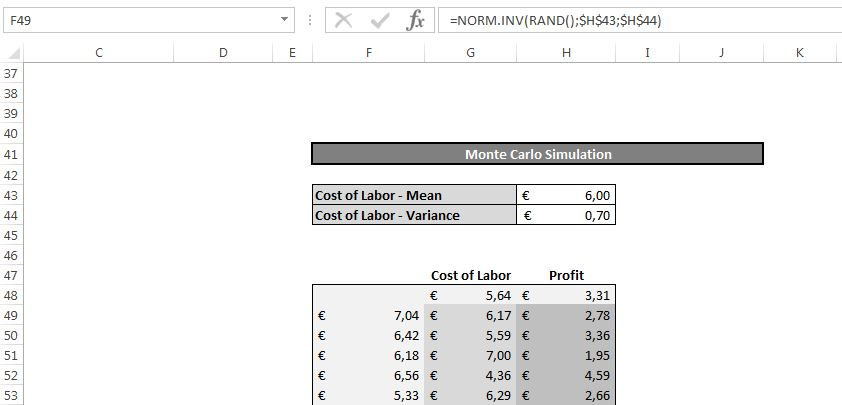What we did in column F is filling our cost of labor according the estimated distribution, but instead of using a fixed probability in the NORM.INV function, we used the RAND() function. And if your number of input values is great enough, in our example we used 1.000 values, your model might - statistically at least - reflect how the future will look like. Let's analyze our profit: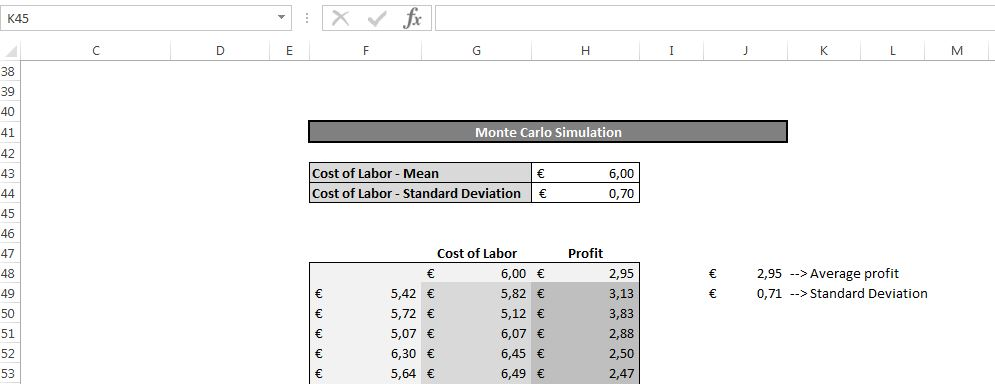As you can see, the average profit is 2,95 ï¿½ with a standard deviation of 0,71 ï¿½. And you can run some further statistics like estimating how much chance you'll have that the profit will be higher than x EUR etc.

Wait a minute, did you need Monte Carlo for that? Of course your average profit is 2,95 ï¿½. If I put 6,00 ï¿½ in my initial model, I could have told you already that my profit would be 2,95 ï¿½...

Good point, but this case is for illustration purposes only. And at least, it proves the model is coherent.

And what if I happen to have multiple input variables for which I know the statistical distribution?

This is indeed the last thing I'd like to share with you today. Suppose all variables - exchange rates and cost of labor - follow a normal distribution, all with their own mean and standard deviation of course. What we'll do is defining this in our model directly: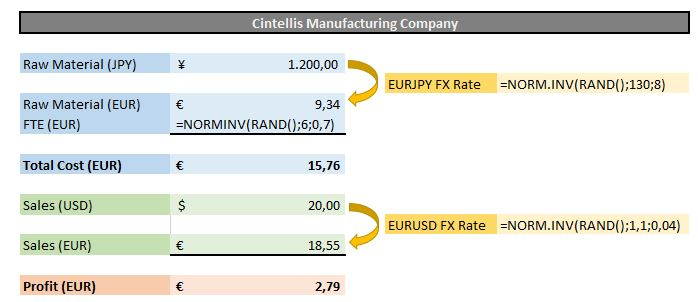Yes but what is now the link with the data tables?

Well, remember that what data tables basically do is iterate. So on each iteration our variables will have another value (there the RAND() function, hence our profit as well, will be different). Again, if we let the data table iterate enough, we'll be able to do some statistical analysis on our results: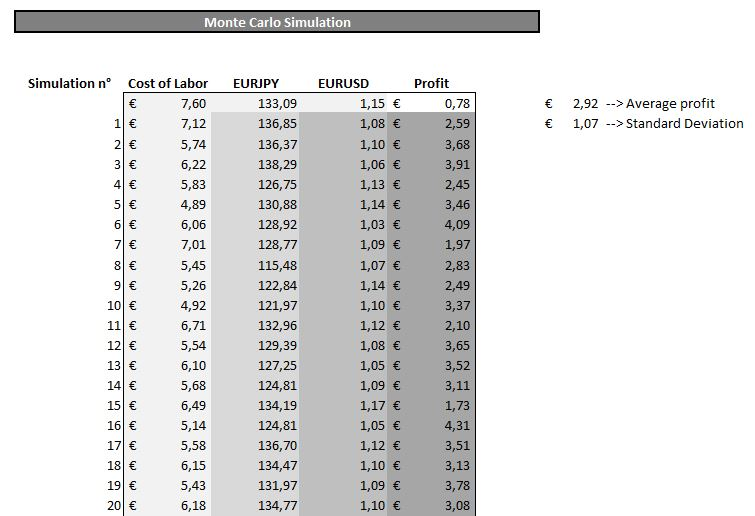The nice thing is, the row or column input cell have become irrelevant, you should just refer to any cell though, otherwise Excel will bother you with an error message.

The irrelevancy comes from the fact that the data table will iterate as many times as rows you selected and per iteration your variables will change, hence it does not need any row or column input.

Good to know, and to remember...

• Monte Carlo is just a model and the results should be used with care. If you don't trust the variability of your inputs, don't trust the model results;

• Don't use just 2 or 10 scenarios. A sufficient number of scenarios should be used to make the variance analysis meaningful;

• If you use a randomly generated number as column or row input cell, every time the data table iterates, the value will change. Hence there will be no link anymore between the input parameter and the measured output. That is why we recommend to add an extra variable to watch in the data table.

Let us surprise you with how much more you can achieve by using Excel and VBA based products.Are you looking for an on the level, reliable and trustworthy company that will surprise you with how much more you can achieve by using Excel and VBA based products? Learn more about our philosophy, products, services or contact us for more info.Flexible, cheap and very short implementation time, discover Numis, our Excel based Cash Management and Management Accounting Software and experience a totally new way to analyse and report on your cash positions.

At Numis, we help you to get more out of your financial data than you thought possible. From enhanced reporting functionalities and detailed analysis to providing enterprise budgeting and forecasting solutions, we will help you to achieve your business objectives. When using Numis, you can share your analysis and strategy with your business partners, suppliers or employees as needed.

Numis is a product from Cintellis bvba & FS&P bvba.